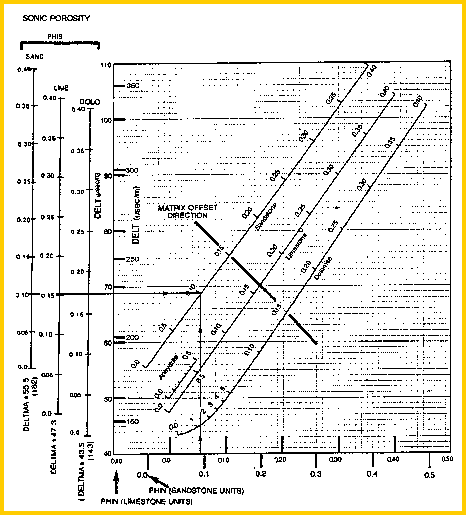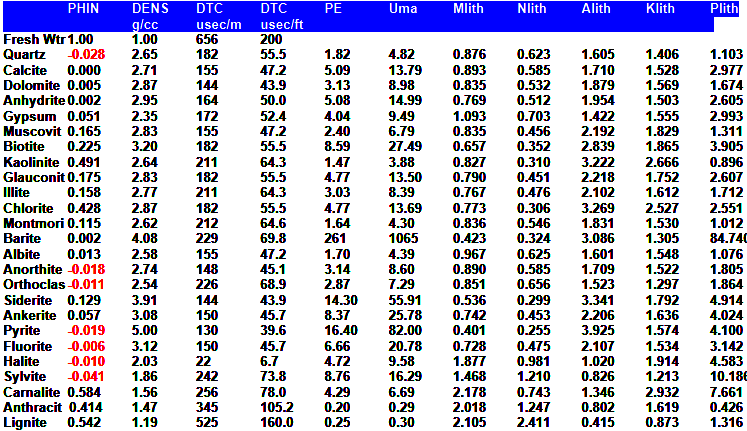Lithology from Matrix TRAVEL TIME
"Slowness" is the new word for sonic or acoustic travel time. The inverse of slowness is speediness or velocity. We continue to use travel time in this Handbook - it's hard to teach old dogs new tricks.

The apparent matrix travel time can be calculated in a similar fashion to the matrix density, again by rearrangement of the response equation.

Calculate Matrix Travel Time:

1: IF Vsh + PHIe < 0.95
2: THEN DTCma = (DTC - PHIe * DTCW - Vsh * DTCSH) / (1 - PHIe - Vsh)
3: OTHERWISE DTCma = DTC

Calculate mineral volumes (relative to each other)
4: Vmin1 = (DTCma - DTC2) / (DTC1 - DTC2)
5: Vmin2 = 1.00 - Vmin1

Calculate mineral volumes (relative to total rock volume)
6: Vrock = (1.0 - Vsh - PHIe)
7: V1 = Vmin1 * Vrock
8: V2 = Vmin2 * Vrock

Where:
DTC = sonic log reading (usec/ft or usec/m)
DTCma = computed matrix travel time (usec/ft or usec/m)
DTC1 = matrix travel time for first mineral (usec/ft or usec/m)
DTC2 = matrix travel time for second mineral (usec/ft or usec/m)
DTCSH = sonic log reading in shale (usec/ft or usec/m)
DTCW = sonic log reading in water (usec/ft or usec/m)
PHIe = effective porosity from any method (fractional)
Vsh = volume of shale (fractional)
Vrock = rock volume (fractional)
Vmin1 = volume of first mineral (fractional)
Vmin2 = volume of second mineral (fractional)
Volumes for lithology track
V1 = volume of first mineral (fractional)
V2 = volume of second mineral (fractional)COMMENTS:
This equation breaks down with high values of PHIe + Vsh, so we set DELTma = DELT when PHIe + Vsh > 0.95.

This model is not very sensitive in carbonates - the matrix density model is better, if data is available.

The matrix travel time can be obtained graphically from the chart below.Sonic neutron crossplot to find DELTmaNUMERICAL EXAMPLE:
1. Assume Sand D in Example 1.
DTC = 300 usec/m
DTCSH = 328 usec/m
Vsh = 0.33
DTCW = 616 usec/m
PHIe = 0.11
DTCma = (300 - 0.11 * 616 - 0.33 * 328) / (1 - 0.11 - 0.33) = 229 usec/m

This value falls in the impossible area and is too high because the sonic log reads high compared to effective porosity found from the density neutron crossplot. If porosity was 0.16, the matrix travel time would be 183 usec/ft (close to the sandstone value).

This is another quality control indicator, and in this example demonstrates a lack of coherence between the sonic and density neutron data, when the matrix, shale and fluid assumption are as given above. Either these parameters, or the log data, or the whole rock model are in error.Sonic Lithology Codes
Lithology codes are more difficult to generate with sonic data then with density data.SlithCode - Sonic Lithology Codes

 DELTma English Metric SlithCode usec/ft usec/m < 41 < 134 -- ---- 41 - 45 134 - 147 DOLO 45 - 49 147 - 160 LIME 49 - 51 160 - 167 ANHY 51 - 58 167 - 190 QRTZ 58 - 65 190 - 213 ---- 65 - 68 213 - 223 SALT 68 - 72 223 - 236 ---- 72 - 76 236 - 249 SYLV 76 - 80 249 - 262 CARN 80 - 120 262 - 393 COAL (only if trigger set) 120 - 124 393 - 406 SULF > 124 > 406 - ---- if Vsh > 0.85 SHLESONIC PARAMETERS